Domain coloring for visualizing complex functions

$f(z)=\dfrac{1}{z^5}+c$

Basic Tools: Real and Imaginary components

With the following tools you just need to enter the real and imaginary components of a complex function $$f(z)=\text{Re}(x, y)+ i \,\text{Im}(x,y).$$

Open the tool by clicking on the image.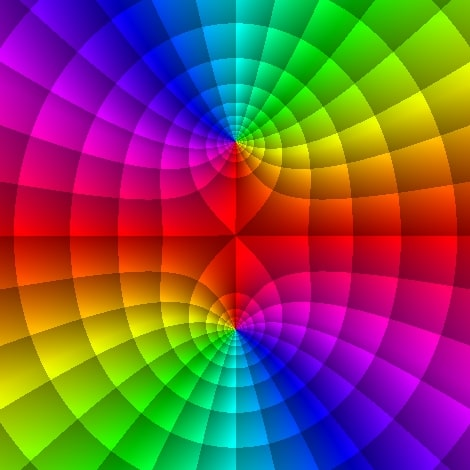HSB enhanced scheme

Plots the level curves of the modulus and phase of $f(z)$.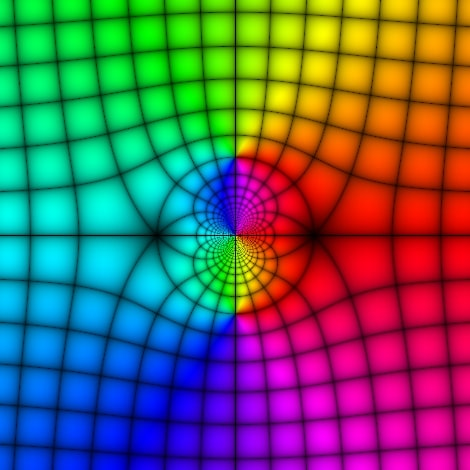HSB enhanced scheme

Plots the level curves of the modulus, imaginary and real components of $f(z)$.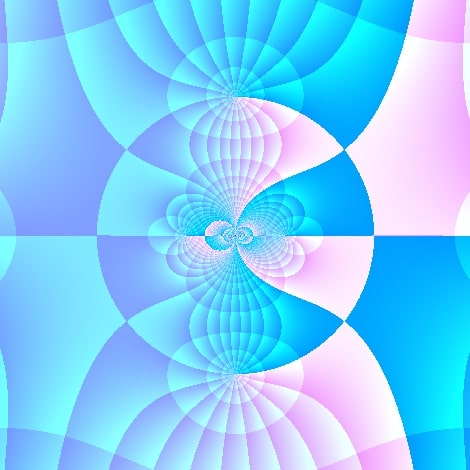RGB enhanced scheme

Plots the level curves of the modulus and phase of $f(z)$.

Advanced Tools: General complex functions

Open the tool by clicking on the image.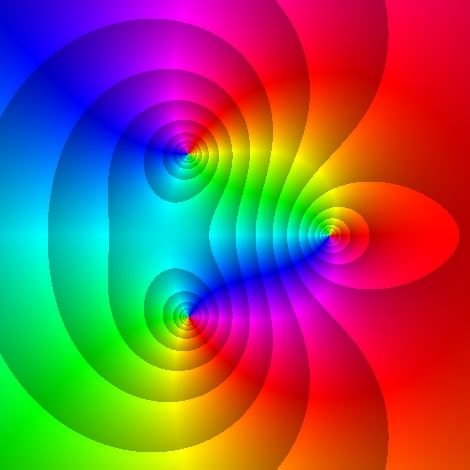HSB enhanced scheme

Plots the level curves of the modulus and phase of $f(z)$.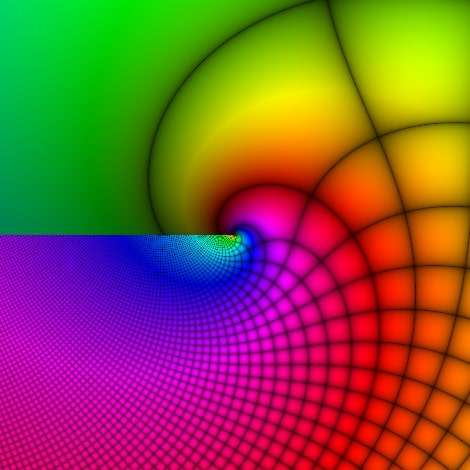HSB enhanced scheme

Plots the level curves of the modulus, imaginary and real components of $f(z)$.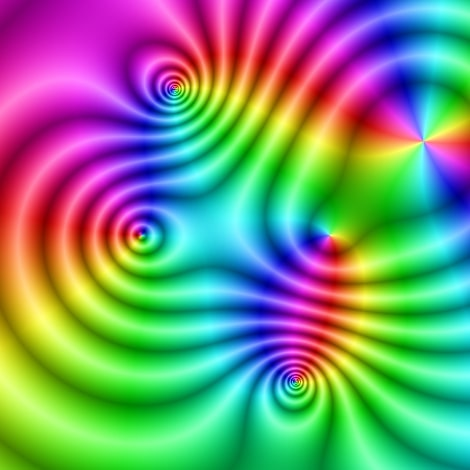HSB smooth enhanced scheme

Plots the modulus of $f(z)$ using a Gradient method.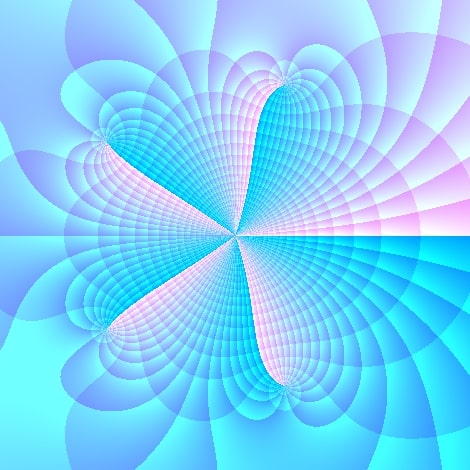HSB enhanced scheme

Plots the level curves of the modulus and phase of $f(z)$.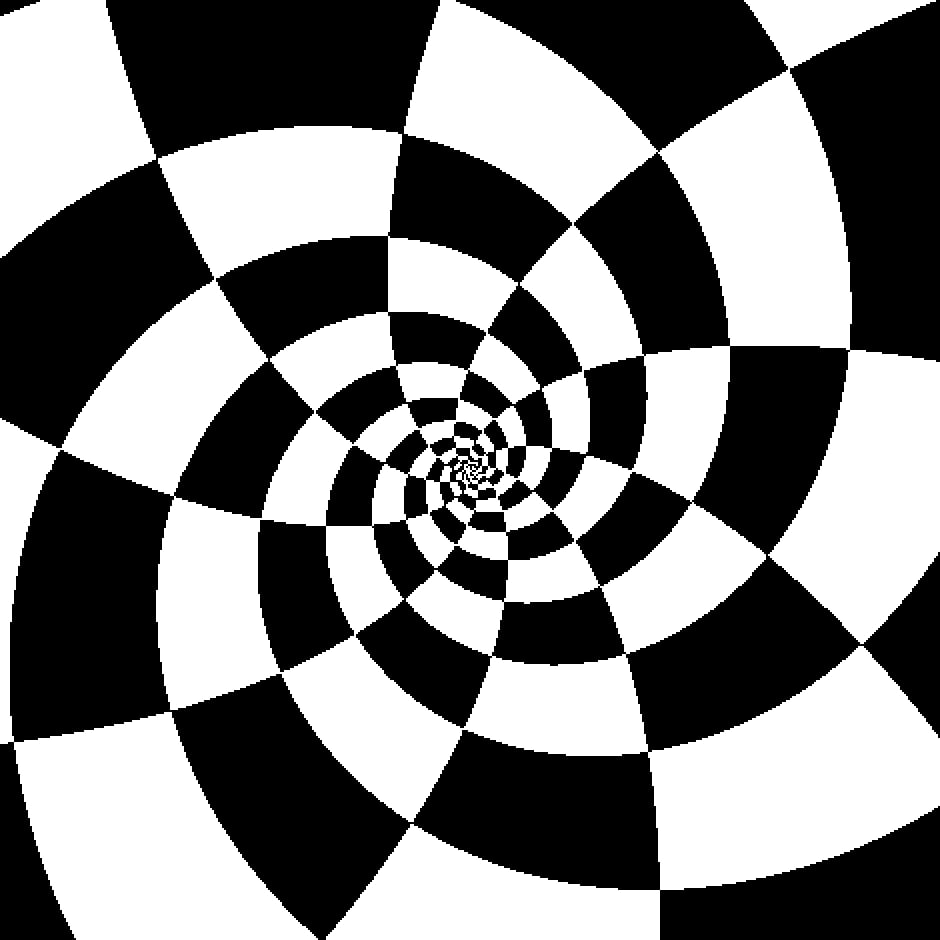Black & White enhanced scheme

Plots phase, modulus, real and imaginary components of $f(z)$.

References

1. Ablowitz, M. J. & Fokas, A. S. (2003). Complex variables: introduction and applications (2nd ed). Cambridge University Press.
2. Breda, A. Trocado, A. & Santos, J. (2013). O GeoGebra para além da segunda dimensão. Indagatio Didactica, 5(1). Recuperado el 12 de junio de 2018
3. Davis, J. E. (2017). Complex Domain Coloring, Available online
4. Crone, L. (s.f) Color graphs of complex functions.
5. Farris, F. (1997). Visualizing complex-valued functions in the plane.
6. Farris, F. A. (1998). Review of Visual Complex Analysis. By Tristan Needham. American Mathematical Monthly 105 (1998), 570–576.
7. Losada Liste, R. (2014). El color dinámico de GeoGebra. Gaceta De La Real Sociedad Matematica Española, 17, 525–547, Madrid.
8. Lundmark, H. (2004). Visualizing complex analytic functions using domain coloring.
9. Needham, T. (1997). Visual Complex Analysis. Oxford University Press, Oxford.
10. Marsden, J. E. & Hoffman, M. J. (1987) Basic Complex Analysis. (2nd ed.) W. H. Freeman and Co, New York.
11. Ponce Campuzano, J. C. (2019) The use of phase portraits to visualize and investigate isolated singular points of complex functions Journal International Journal of Mathematical Education in Science and Technology. 50(7), pp. 999-1010.
12. Wegert, E. (2010). Phase diagrams of meromorphic functions. Comput. Methods Funct. Theory 10, 639-661.
13. Wegert, E. (2012). Visual Complex Functions: An introduction with phase portraits. New York: Springer Basel.
14. Wegert, E. (2016). Visual exploration of complex functions. In T. Qian and L. R. Rodino (eds.), Mathematical analysis, probability and applications – Plenary Lectures SPMS (Vol. 177. pp. 253–279). Switzerland: Springer International Publishing.
15. Wegert, E. & Semmler, G. (2011). Phase plots of complex functions: a journey in illustration. Notices American Mathematical Society, 58, 768-780.
16. Wikipedia: Domain Coloring Recuperado